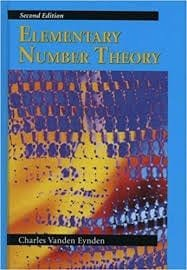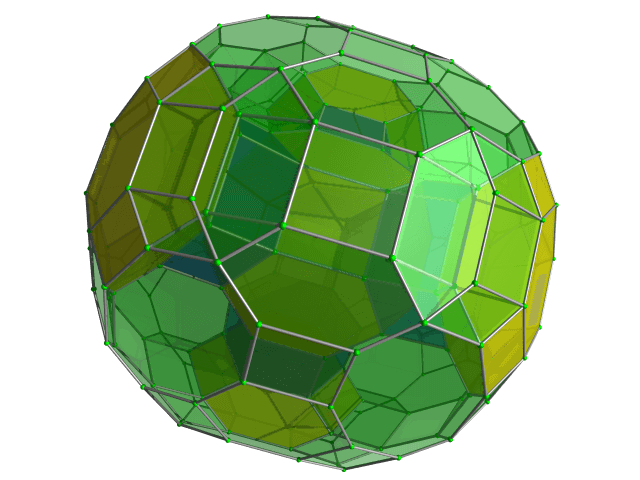# Number Theory  Module Level 2

#### When

• Every Saturday
• 10 AM to 11:30 AM I.S.T.

#### Where and who can attend

• Live, Online
• Only internal students at Cheenta may attend.

#### What will we learn?

Number theory (or arithmetic or higher arithmetic in older usage) is a branch of Pure Mathematics devoted primarily to the study of the integers and integer-valued functions. German mathematician Carl Friedrich Gauss (1777–1855) said, “Mathematics is the queen of the sciences—and number theory is the queen of mathematics.” Number theorists study Prime numbers as well as the properties of objects made out of integers (for example, Rational Numbers) or defined as generalizations of the integers (for example, algebraic integers). Number theory seeks to understand the properties of integer systems in spite of their apparent complexity..

#### This week...

The Chinese Remainder Theorem – Manifold Interpretations.

# Faculty#### Swarnabja Bhaumik

Faculty, Alumni at Cheenta

pursuing B.Tech. Computer Science from the University of Calcutta

Research Interest: Machine Learning, Artificial Intelligence

# Books and reference#### Elementary Number theory.

by Charles Vanden Eynden

# How to join?